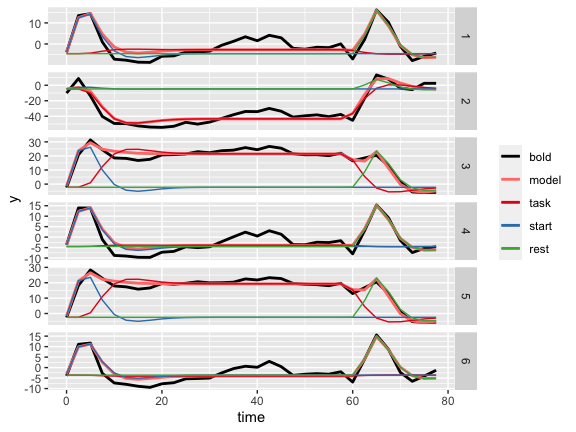# Analysis steps used in the flanker study

#### 2023-02-15

Here, we present the analysis steps that were used for the evaluation of the autohrf package based on the flanker study discussed in the paper Purg, N., Demšar, J., & Repovš, G. (2022). autohrf – an R package for generating data-informed event models for general linear modeling of task-based fMRI data. Frontiers in Neuroimaging.

We start the analysis by loading relevant libraries and the fMRI data collected during the flanker task.

# libraries
library(autohrf)
library(dplyr)
##
## Attaching package: 'dplyr'
## The following objects are masked from 'package:stats':
##
##     filter, lag
## The following objects are masked from 'package:base':
##
##     intersect, setdiff, setequal, union
library(ggplot2)
library(magrittr)

df <- flanker
head(df)
##   roi    t          y
## 1   1  0.0 -3.9917023
## 2   1  2.5 13.4798112
## 3   1  5.0 14.3976919
## 4   1  7.5  0.9602429
## 5   1 10.0 -7.2285326
## 6   1 12.5 -7.7171224

The loaded data frame has 192 observations, which correspond to the fMRI measurements for six clusters of brain areas over 32 time points during the flanker task trial. The fMRI data has been averaged for individual voxels within each region of interest (ROI) and additionally within each ROI cluster, across task trials, and across 30 participants. Each observation has three variables (roi, t, and y), where roi denotes the ROI cluster, t the time stamp and y the value of the BOLD signal.

To visually inspect the data we plot the average activity during a single trial of the flanker task for all six ROI clusters.

# visualize the data
df %>%
mutate(roi = factor(roi)) %>%
ggplot(aes(x = t, y = y, color = roi)) +
geom_line() +
facet_grid(roi ~ .)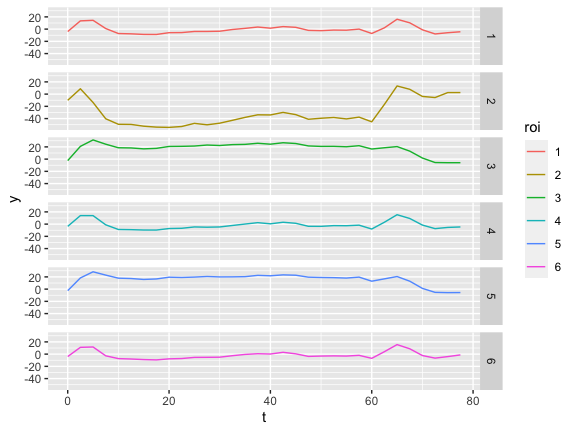Next, we want to find the optimized event model to be used in general linear modeling of the flanker fMRI data. For that purpose, we prepare two sets of model constraints that are going to be used in the automated parameter search, one with stricter constraints and the other with more permissive constraints.

# setup model constraints
modelS <- data.frame(event        = c("start", "task", "rest"),
start_time   = c(      0,       0,     60),
end_time     = c(      2,      60,     65),
min_duration = c(    0.1,      55,    0.1))

modelP <- data.frame(event        = c("start", "task", "rest"),
start_time   = c(      0,       0,     55),
end_time     = c(      5,      65,     65),
min_duration = c(    0.1,      50,    0.1))

# join models
models <- list(modelS, modelP)

We run the automated parameter search using the autohrf function. We additionally define weights for each ROI cluster, which reflect the proportion of all ROIs in a specific cluster. In that way, we put more weight on how the model fits a particular ROI cluster compared to the rest of ROI clusters.

# optimization settings
tr         <- 2.5
population <- 100
iter       <- 1000
hrf        <- "spm"

# cluster weights
w <- data.frame(roi    = c(   1,    2,    3,    4,    5,    6),
weight = c(0.14, 0.06, 0.25, 0.15, 0.22, 0.19))

# run optimization (to speed vignette building we load results from a previous autohrf run)
# autofit <- autohrf(df, models, tr=tr, hrf=hrf, population=population, iter=iter, roi_weights=w)
autofit <- flanker_autofit

Based on the results obtained with the autohrf function we examine the convergence of model fitness using the plot_fitness function, extract estimated model parameters using the get_best_models function, and inspect how well the models fit the measured BOLD signal using the evaluate_model and plot_model functions.

# check the convergence of model fitness
plot_fitness(autofit)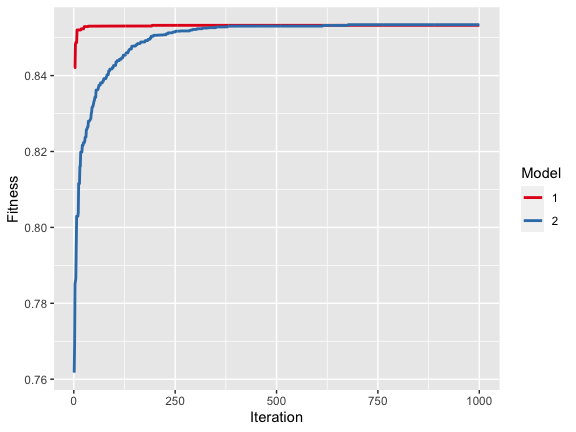# return derived parameters
best_models <- get_best_models(autofit)
##
## ----------------------------------------
##
## Model 1
##
## Fitness:  0.8532481
##
##   event  start_time  duration
## 1 onset  0.05963566  0.102654
## 2 block  3.00067156 54.698517
## 3  rest 60.05001943  2.526290
##
## ----------------------------------------
##
## ----------------------------------------
##
## Model 2
##
## Fitness:  0.8534121
##
##   event start_time   duration
## 1 onset   0.000000  0.1865176
## 2 block   2.948766 54.7415242
## 3  rest  60.050583  2.5252960
##
## ----------------------------------------
# evaluate models
emS <- evaluate_model(df, best_models[], tr=tr, hrf="spm", roi_weights=w)
##
## Mean R2:  0.8444376
## Median R2:  0.829568
## Min R2:  0.7611278
## Weighted R2:  0.8540102
##
## Mean BIC:  182.2334
## Median BIC:  179.871
## Min BIC:  150.9915
## Weighted BIC:  174.0449
emP <- evaluate_model(df, best_models[], tr=tr, hrf="spm", roi_weights=w)
##
## Mean R2:  0.8447613
## Median R2:  0.830379
## Min R2:  0.7615092
## Weighted R2:  0.8541756
##
## Mean BIC:  182.2039
## Median BIC:  179.793
## Min BIC:  151.1411
## Weighted BIC:  174.0697
# plot model fits
plot_model(emS)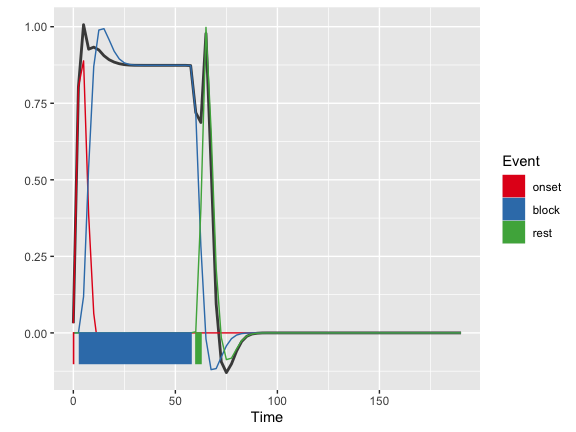plot_model(emP)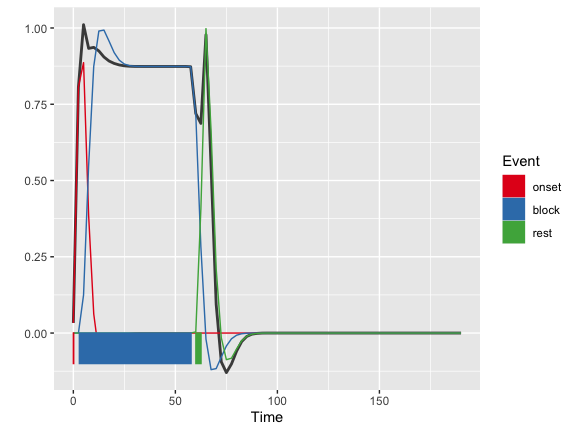# plot model fits for individual ROI clusters
plot_model(emS, by_roi = TRUE, nrow=6)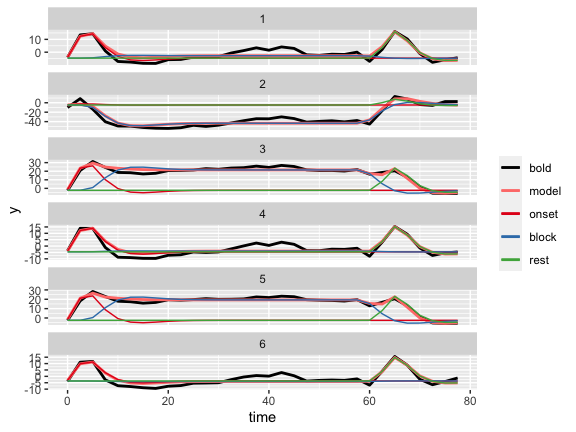plot_model(emP, by_roi = TRUE, nrow=6)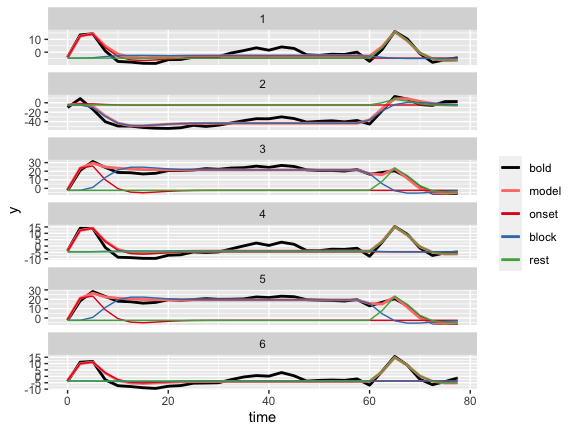We then perform a focused evaluation of event models with different levels of complexity. Specifically, we evaluate two models based on theoretical assumptions of the task structure underlying BOLD responses, one with a single event predictor and one with three event predictors. We also evaluate one model with three event predictors that were optimized using the automated parameter search.

# prepare models
start_time = c(0),
duration   = c(60))

model2 <- data.frame(event      = c("task", "start", "rest"),
start_time = c(     0,       0,     60),
duration   = c(    60,       1,      1))

model3 <- data.frame(event      = c("task", "start", "rest"),
start_time = c(  3.00,    0.00,  60.00),
duration   = c( 54.70,    0.18,   2.53))

# model settings
tr  <- 2.5
hrf <- "spm"

# evaluate models
em1 <- evaluate_model(df, model1, tr=tr, hrf=hrf, roi_weights = w)
##
## Mean R2:  0.390662
## Median R2:  0.3772886
## Min R2:  0.05357616
## Weighted R2:  0.3877339
##
## Mean BIC:  219.7373
## Median BIC:  216.7965
## Min BIC:  209.4884
## Weighted BIC:  216.0819
em2 <- evaluate_model(df, model2, tr=tr, hrf=hrf, roi_weights = w)
##
## Mean R2:  0.793958
## Median R2:  0.793676
## Min R2:  0.6802623
## Weighted R2:  0.7931015
##
## Mean BIC:  193.5461
## Median BIC:  190.4257
## Min BIC:  181.5361
## Weighted BIC:  189.5171
em3 <- evaluate_model(df, model3, tr=tr, hrf=hrf, roi_weights = w)
##
## Mean R2:  0.8444939
## Median R2:  0.8297674
## Min R2:  0.7602191
## Weighted R2:  0.8540895
##
## Mean BIC:  182.2592
## Median BIC:  179.7613
## Min BIC:  151.229
## Weighted BIC:  174.0786
# plot model fits
plot_model(em1, by_roi=TRUE, ncol=1) + facet_grid(roi ~ ., scales="free_y")
## Warning in brewer.pal(length(events), "Set1"): minimal value for n is 3, returning requested palette with 3 different levels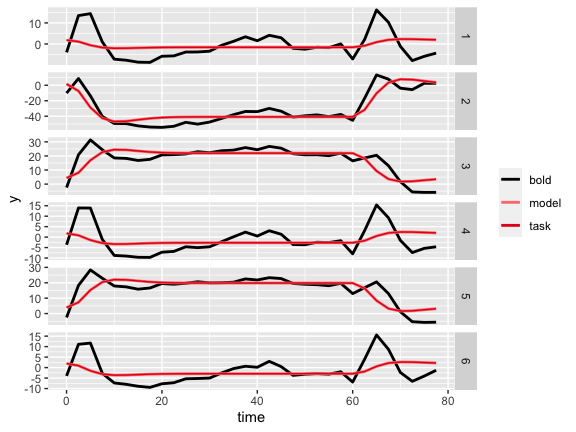plot_model(em2, by_roi=TRUE, ncol=1) + facet_grid(roi ~ ., scales="free_y")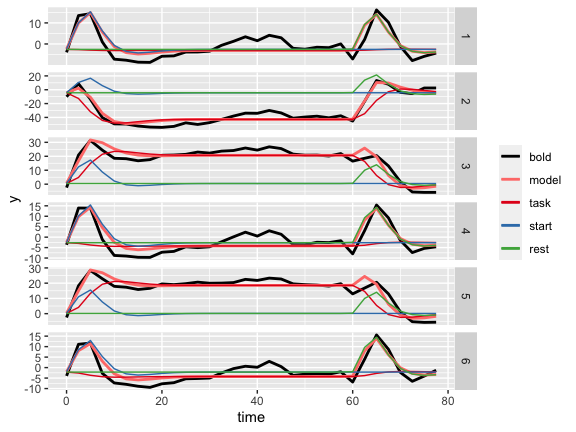plot_model(em3, by_roi=TRUE, ncol=1) + facet_grid(roi ~ ., scales="free_y")